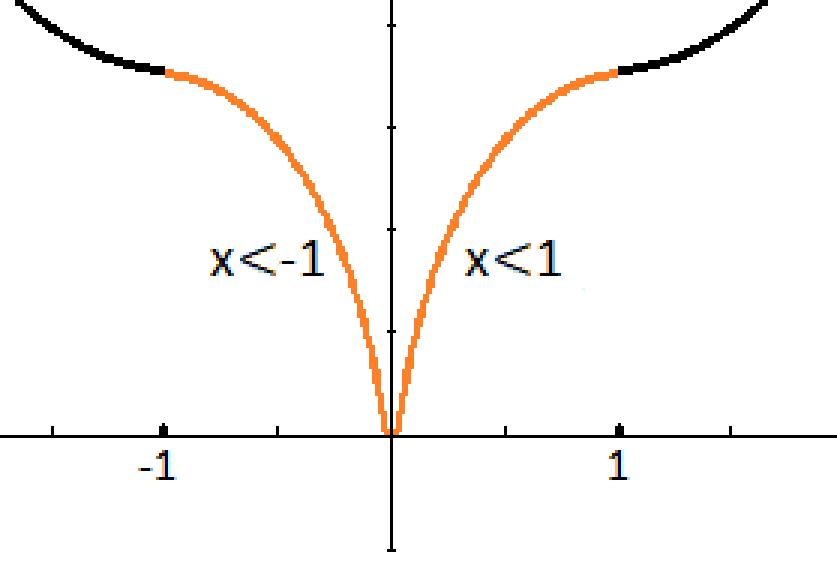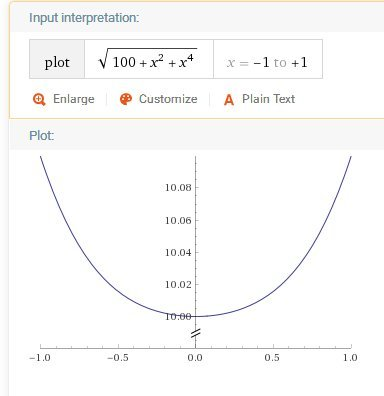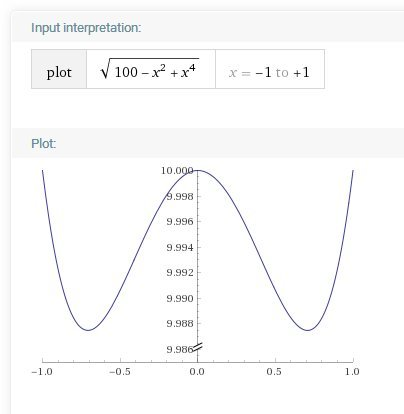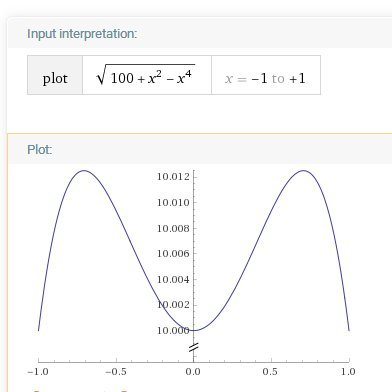Jump to content

# Anharmonic Oscillator

## Recommended Posts

• Replies 276
• Created
• Last Reply

#### Popular Posts

The gauge groups I mentioned are not specifically the harmonic oscillator. QFT accounts for the quantum harmonic oscillator in its gauge groups but that isn't the full story.  https://www.google.

Sigh no you have to recognize that harmonic, inharmonic and anharmonic are descriptives of the characteristics of the oscillator.  Here is how classical harmonic oscillator is described.  A

Be careful of such bold statements for example try to define that statement above to the Higgs coupling constants. First ask yourself the function of a coupling constant in regards to mass. (Hint

#### Posted Images

10 hours ago, Strange said:

It would just be seen as a particle that moves very fast.

Ah ok. And in math what does this give?

11 hours ago, Strange said:

("with our eyes"? We can't see single points, even if they are stationary. I am assuming you are talking about making measurements with appropriate tools.)

Yes, with the eyes. How to observe other than the microscope, telescope or with the naked eye?

What does this sentence mean, and why: We can't see single points, even if they are stationary

Even if they are stationary ?

##### Share on other sites
8 hours ago, Kartazion said:

Ah ok. And in math what does this give?

I don't know what you mean. Use the standard equations of motion (kinematics), presumably. Although there are interesting effects like Cherenkov radiation from particles moving faster than light.

Quote

Yes, with the eyes. How to observe other than the microscope, telescope or with the naked eye?

What does this sentence mean, and why: We can't see single points, even if they are stationary

Even if they are stationary ?

You can't see electrons, neutrinos, muons, etc with the naked eye. They are all point particles (ie with zero size). You can't even see protons or neutrons, or even atoms with the naked eye.

##### Share on other sites
6 minutes ago, Strange said:

I don't know what you mean. Use the standard equations of motion (kinematics), presumably. Although there are interesting effects like Cherenkov radiation from particles moving faster than light.

$$m \frac{d^2 x}{dt^2}- K x+AKx^3=0$$

The cyan line corresponds to the kinetic Energy http://ressources.univ-lemans.fr/AccesLibre/UM/Pedago/physique/02/meca/oscilanhar.html

Going faster than light is an option that is not mandatory.

14 minutes ago, Strange said:

You can't see electrons, neutrinos, muons, etc with the naked eye. They are all point particles (ie with zero size). You can't even see protons or neutrons, or even atoms with the naked eye.

I'm talking about visible particle (you know the yellow dot that oscillates from the previous link).
I'm not talking about alternating an electron or other subatomic particles. Just a small mass visible to the naked eye.

##### Share on other sites
9 hours ago, Kartazion said:

Ah ok. And in math what does this give?

What do you mean what does the math give?

What do you think it gives ? (I already told you it gives you the answer to your original question)

What more do you want?

Hint what happens if you put x = 0 into that equation?
(I also suggest you replace dx/dt with v for velocity)

##### Share on other sites
On 11/25/2019 at 10:39 PM, studiot said:

Then the equation becomes

\frac{{{d^2}x}}{{d{t^2}}} + ax + b{x^3} = 0

Hello studio.

Yes, I'm still thinking about the equation.
What is b?

##### Share on other sites
2 minutes ago, Kartazion said:

Hello studio.

Yes, I'm still thinking about the equation.
What is b?

This is ridiculous.

Why don't you just answer a straightforward question?

What happens in the equation

${v^2} = C - a{x^2} - \frac{1}{2}b{x^4}$

if if we put x=0 ?

Note you may need to refresh the page once to see the result of the mathml formatting.

##### Share on other sites
5 minutes ago, studiot said:

What happens in the equation

2=Cax212bx

if if we put x=0 ?

The result is equal to C. The mass is then found at 0 on the x axis.

##### Share on other sites
1 hour ago, studiot said:

Indeed, so what does this mean physically?

Is this a maximum or a minimum?

It is the mass at rest and it depends on the spring at rest to locate 0 on the axis, so I would say towards the middle, at the intersection of y.

##### Share on other sites
26 minutes ago, Kartazion said:

It is the mass at rest and it depends on the spring at rest to locate 0 on the axis, so I would say towards the middle, at the intersection of y.

How can it be?

What about velocity? This is after all what you asked about.

Yes, x = 0 means the place where the y axis crosses the x axis.

But what are you plotting on the y axis?

Again I ask what about velocity?

If the particle (call it 'the particle' not 'the mass' to avoid confusion with the mass term, m,  in the original equation) is oscillating what is it doing at x=0?

##### Share on other sites
21 minutes ago, studiot said:

How can it be?

What about velocity? This is after all what you asked about.

Yes, x = 0 means the place where the y axis crosses the x axis.

But what are you plotting on the y axis?

Again I ask what about velocity?

If the particle (call it 'the particle' not 'the mass' to avoid confusion with the mass term, m,  in the original equation) is oscillating what is it doing at x=0?

I did'nt understand everything. But the speed at 0 is at its max, while the potential energy is at its minimum?
The y-axis precisely represents the potential energy submitted to the particle?

##### Share on other sites
27 minutes ago, Kartazion said:

I did'nt understand everything. But the speed at 0 is at its max, while the potential energy is at its minimum?
The y-axis precisely represents the potential energy submitted to the particle?

Good we are getting somewhere.

Yes v2 = C gives the velocity of the particle at x = 0; and it is a maximum.

Now I hope you know and understand that

1) A single oscillator does not make a wave

2) This equation is not a wave equation.

3) The velocity of a wave is not the same as the velocity of the particles.

So this equation gives us the velocity of the particle not a wave.

Of course since this is the maximum value this is the place where the particle would first reach the speed of light.
(Which is what you asked about)

If we write v0 = √C and the whole equation as

$v = \sqrt {{{\left( {{v_0}} \right)}^2} - a{x^2} - \frac{1}{2}b{x^4}}$

we have an equation for the velocity a a function of the displacement, x.

Because the square root must not be of a negative number, this places some conditions on possible values of a and b.

Edited by studiot
##### Share on other sites
On 11/29/2019 at 2:31 PM, studiot said:

1) A single oscillator does not make a wave

2) This equation is not a wave equation.

3) The velocity of a wave is not the same as the velocity of the particles.

So this equation gives us the velocity of the particle not a wave.

Yes it's really logical. But I'm wondering why you tell me so, because it takes me to the Schrödinger equation or maybe even Maxwell. Is there a report?

On 11/29/2019 at 2:31 PM, studiot said:

If we write v0 = √C and the whole equation as

v=(v0)2ax212bx4

we have an equation for the velocity a a function of the displacement, x.

Everything is therefore based on v as a function of x.

But I have a very silly question:
If (v0)² is different from v² then what is the result of (v0)² if v=2 ?

##### Share on other sites
1 minute ago, Kartazion said:

But I have a very silly question:
If (v0)² is different from v² then what is the result of (v0)² if v=2 ?

I'm sorry but it is a very silly question.

Please explain your level in mathematics This is very important since I am assuming you not only know simple calculus such as the meaning of dx/dt but also more difficult stuff since you posted a very difficult differential equation.

What do you understand the constant (v0)2 to mean ?

What do you understand the variable v to mean ?

4 minutes ago, Kartazion said:

Everything is therefore based on v as a function of x.

Not quite, especially since the equation you posted does not contain v (or dx/dt).

##### Share on other sites
4 minutes ago, studiot said:

Please explain your level in mathematics This is very important since I am assuming you not only know simple calculus such as the meaning of dx/dt but also more difficult stuff since you posted a very difficult differential equation.

Beginner.

6 minutes ago, studiot said:

What do you understand the constant (v0)2 to mean ?

I really do not know.

v0 = √C  = x at zero = maximum velocity

The constant (v0)2 is the maximum Velocity squared?

14 minutes ago, studiot said:

What do you understand the variable v to mean ?

The value metre per second?

Now I'm seeing for the Leibniz's notation.

##### Share on other sites
3 hours ago, Kartazion said:

I really do not know.

v0 = √C  = x at zero = maximum velocity

The constant (v0)2 is the maximum Velocity squared?

I know I was wrong. Because C is different of √C.
So if v²=C when x=0 then √C also gives the root for x is √x

I only know f(x) and I understand for the time being the infinitesimal interval of dx/dt.

I have not yet understood v0. Maybe you would have a link that explains it?

Edited by Kartazion
spelling mistake
##### Share on other sites

I start from the principle where v0 is the velocity when x=0. But since I do not know much, we'll see.

On 11/29/2019 at 2:31 PM, studiot said:

If we write v0 = √C and the whole equation as

$$v = \sqrt {{{\left( {{v_0}} \right)}^2} - a{x^2} - \frac{1}{2}b{x^4}}$$

However I have another problem to raise.

I know very well that a and b are constants. But it would be necessary for a to become a variable, or to use a second constant named e linked to a if a remains a constant.

Indeed I wish to use these conditions in the equation:
ax if x⩾1
ax if x⩾-1
and
ex if x<1
ex if x<-1

This allows me to force the acceleration of the particle when x tends to 0.
Here is the desired curve:I would like to keep the form of the equation except that when ax approaches ~1 (0.999...) for x, then the constant a changes its value (a becomes e)
Maybe it should be two constants related to extrapolated values written as eax?
Or is there another solution?

Sorry for not being able to express myself better.

Edited by Kartazion
LaTeX
##### Share on other sites
2 hours ago, studiot said:

Do you want you oscillator to have infinite velocity at x = 0 ?

That is what you seem to have drawn.

Good evening studio.

Let's say really fast, but not infinite, because if this velocity is infinite at x = 0 we can not have duly determined velocity.

The particle moves according to x and goes from for example, from -0.01 to 0.01 quickly. On the x-axis -0.01 and 0.01 being determined velocities for the particle.
So its acceleration and velocity is determined at x = 0 if the particle stay in passing half a nanosecond for example.

How to go from a determined speed (-0.01 for x) to infinity (x = 0) and then return to a determined speed (0.01 for x)?

If the velocity at x = 0 is infinite, or not, does this change the shape of the equation between the two choices?

##### Share on other sites

Because the curvature of the two yellow lines you have drawn are opposite, the cusp they form must pass through a vertical line whcih goes to negative infinity.

This line is at x = 0 which is why I said the velocity there is infinite.

##### Share on other sites

I have re-drawn the curve to avoid infinity.##### Share on other sites

But apparently what I drew is always a cusp. So let's do it with a cusp.

Within possible limits, how would the equation $$v = \sqrt {{{\left( {{v_0}} \right)}^2} - a{x^2} - \frac{1}{2}b{x^4}}$$ be with a cusp in addition, when x becomes x<-1 and x<1 ?

Edited by Kartazion
LaTeX
##### Share on other sites
6 hours ago, Kartazion said:

But apparently what I drew is always a cusp. So let's do it with a cusp.

Within possible limits, how would the equation

v=(v0)2ax212bx4

be with a cusp in addition, when x becomes x<-1 and x<1 ?

You are not plotting the described function it looks nothing like your cusp.

Here are plots with a and b both positive, a negative be positive, b negative and a positive.##### Share on other sites

Your plotting software looks really good. Thank you for that clarification.

So, is it possible to insert a cusp parametric equation of the original equation? I think no, because I do not see how to reverse the tendency of y to -y without changing the original equation.
I also thought of a partial differential equation (PDE), but I do not know if we can create a cusp with a PDE.

## Create an account or sign in to comment

You need to be a member in order to leave a comment

## Create an account

Sign up for a new account in our community. It's easy!

Register a new account

## Sign in

Already have an account? Sign in here.

Sign In Now

×

• #### Activity

• Leaderboard
×
• Create New...

## Important Information

We have placed cookies on your device to help make this website better. You can adjust your cookie settings, otherwise we'll assume you're okay to continue.